# Important Questions for Class 12 Chemistry Chapter 4 - Chemical Kinetics

Class 12 chemistry important questions with Answers are provided here for chapter 4 Chemical Kinetics. These important questions are based on CBSE board curriculum and correspond to the most recent Class 12 chemistry syllabus. By practicing these Class 12 important questions, students will be able to quickly review all of the ideas covered in the chapter and prepare for the Class 12 Annual examinations as well as other entrance exams such as NEET and JEE.

Download Class 12 Chemistry Chapter 4 – Chemical Kinetics Important Questions with Answers PDF by clicking on the button below.

## Class 12 Chemical Kinetics Important Questions with Answers

Q1. State a condition under which a bimolecular reaction is kinetically a first order reaction.

This is possible in case one of the reactants is taken in large excess so that its concentration changes very slightly in the reaction. The reaction is a pseudo first-order reaction. For example, hydrolysis of an ester in an acidic medium.

Q2. Write the rate equation for the reaction 2A + B → C if the order of the reaction is zero.

The rate equation for the reaction 2A + B → C in the zero order reaction is

Rate = k [A]0 [B]0 = k

3. How can you determine the rate law of the following reaction?

2NO(g) + O₂(g) → 2NO₂ (g)

The rate law for the reaction can be determined experimentally. Firstly, the concentration of one of the reactants let O2 is taken in large excess and the reaction rate is determined with respect to NO (g). Then the other reactant NO is taken in large excess and the reaction rate is determined with respect to O2. The rate law expression for the reaction may be expressed as

Rate = k [NO]x [O2]y

Where x and y are actual coefficients of the reaction.

Q4. For which type of reactions, order and molecularity have the same value?

Order and molecularity have the same value for elementary reactions that are taking place in a single step.

Q5. In a reaction, if the concentration of reactant A is tripled, the rate of reaction becomes twenty-seven times. What is the order of the reaction?

For the reaction, rate (r) = k [A]n ……..(1)

According to the given date rate (27r) = k [3A]n ………(2)

Divided equation (2) by (1) , (27r)/(r) = k [3A]n/ k [A]n ⇒ 27 = 3n ⇒ n = 3

The order of the reaction is 3.

Q6. Derive an expression to calculate the time required for completion of a zero order reaction.

From the zero order reaction we know [A] = [A]0 – k t

For the completion, [A] = 0, therefore t = [A]0 / k

Q7. For a reaction A + B → Products, the rate law is – Rate = k [A][B]3/2 Can the reaction be an elementary reaction? Explain.

In an elementary reaction, the number of atoms or ions which are colliding to react is known as molecularity. Had the reaction been of elementary nature (single step reaction) the order of reaction with respect should have been 1 but it is 3/2 i.e. fractional. This shows that the reaction is of complex nature and is not an elementary reaction.

Q8. For a certain reaction a large fraction of molecules has energy more than the threshold energy, yet the rate of reaction is very slow. Why?

The reaction rate generally depends on two factors : orientation factor and energy factor. Apart from possessing energy equal to threshold energy. The reacting species must be properly oriented at the time of collision. In the reaction under observation, the orientation effect is not proper. Therefore, effective collisions are not much as expected. The reaction is therefore, a slow reaction.

Q9. For a zero order reaction will the molecularity be equal to zero? Explain.

No, the molecularity for the reaction can neither be zero nor fractional.

Q10. For a general reaction A B, the plot of concentration of A vs time is given in Fig. Answer the following questions on the basis of graph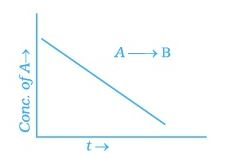(a) What is the order of the reaction ?

(b) What is the slope of the curve?

(c) What is the unit of the rate constant?

(i) From the graph of the concentration of A verse to show the equation of zero order reaction i.e [A] = [A]0 – kt , here the slope is negative.

(ii) The slope of the curve is -k

(iii) The unit of the rate constant = mol L-1 s-1

Q11. The reaction between H₂(g) and O₂(g) is highly feasible yet allowing the gasses to stand at room temperature in the same vessel does not lead to the formation of water. Explain.

The reaction between H₂(g) and O₂(g) is highly feasible yet allowing the gasses to stand at room temperature in the same vessel does not lead to the formation of water because the activation energy for the reactants is very high at room temperature and it is not readily available.

Q12. Why does the rate of a reaction increase with a rise in temperature?

With rise in temperature, the energy possessed by the reacting species increases. As a result, more species are in a position to cross the activation barrier. Therefore the reaction rate increases with a rise in temperature.

Q13. Oxygen is available in plenty in air yet fuels do not burn by themselves at room temperature. Explain.

The activation energy for the combustion reactions of fuel is generally very high. This is not available at room temperature. The fuels as such are not in a position to burn or undergo combustion at room temperature.

Q14. Why is the probability of reaction with molecularity higher than three very rare?

Molecularity of a reaction is defined as the number of the reacting molecules/species that are colliding simultaneously. As the number increases, the chances of their simultaneous collision decreases. This means that it is very little probable that more than three reacting molecules may collide simultaneously. In other words, the probability of reactions with molecularity more than three is very rare.

Q15. Why does the rate of any reaction generally decrease during the course of the reaction?

The rate of any reaction is proportional to the concentration of the reactants. With the progress of the reaction, the concentration decreases gradually and so does the rate of reaction.

Q16. Thermodynamic feasibility of the reaction alone cannot decide the rate of the reaction. Explain with the help of one example.

Many chemical reactions are thermodynamically feasible which means that the enthalpy of reaction (ΔrH) is negative. Still they do not occur. Actually, the activation energy is another factor which influences the reaction velocity. In case, the reacting molecules do not have sufficient activation energy, their collisions will go waste without resulting in products.

Q17. Why in the redox titration of KMnO4 vs oxalic acid, do we heat the oxalic acid Answer before starting the titration?

The reaction is very slow at room temperature. By raising the temperature, the reaction rate increases. Normally in this reaction, KMnO4 acidified with dilute H₂SO4 reacts with oxalic acid at a temperature of about 50°C.

Q18. Why can’t the molecularity of any reaction be equal to zero?

Molecularity of any reaction is given as the number of the reactant molecules or species that are colliding simultaneously in the elementary reaction. A minimum of one reactant is needed to initiate a chemical reaction. Therefore, the molecular mass of the reaction cannot be zero.

Q19. Why is molecularity applicable only for elementary reactions and order is applicable for elementary as well as complex reactions?

Elementary reactions proceed in a single step while complex reactions generally take place in many steps. In multiple step reactions, each step has its own molecularity and the molecularity of these steps are not added up. Therefore, complex reactions have no molecularity. On the other hand, in a complex reaction the order as determined for the slowest step, also called rate determining step, represents the order for the entire reaction. Hence, we may conclude that molecularity is applicable only to the single step reactions whereas order applies to both elementary and complex reactions.

Q20. Why can we not determine the order of a reaction by taking into consideration the balanced chemical equation?

The sum of the stoichiometric coefficients of the reacting species in a balanced chemical equation may or may not represent its order. This is possible only for elementary reactions which proceed in a single step. In multiple step reactions, the coefficients of the reacting species involved in the rate law determine the order of the reaction. For example,

KClO3 + 6FeSO4 + 3H2SO4 → KCl + 3Fe2(SO4)3 + 3H2O

The sum of the stoichiometric coefficients in the reaction is ten. But the reaction has been found to be of second order. This means that it is a multistep reaction and in the slowest or the rate-determining step the sum of the coefficients of the reacting species involved is only two.

### Matching Type Questions

Note: In the following questions match the items of Column I with the appropriate item given in Column II.

Q1. Match the graph given in Column I with the order of reaction given in Column II. More than one item in Column I may link to the same item in Column II.

Column I Column II
(a)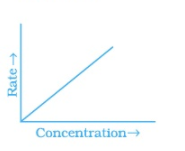(i) 1st order
(b)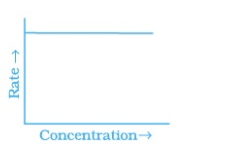(ii) Zero order
(c)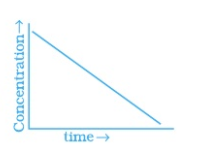(d)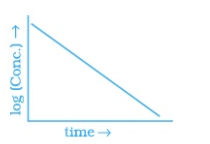(a) – (i) ; (b) – (ii); (c) – (ii); (d) – (i)

Q2. Match the statements given in Column I and Column II

Column I Column II
(a) Catalyst alters the rate of reaction (i) cannot be fraction or zero
(b) Molecularity (ii) proper orientation is not there always
(c) Second half life of first order reaction (iii) By lowering the activation energy
(d) e-Ea/RT (iv) is same as the first
(e) Energetically favorable reactions are sometimes slow (v) total probability is one
(f) Area under the Maxwell Boltzman curve is constant (vi) refers to the fraction of molecules with energy equal to or greater than activation energy

(a) – (iii) ; (b) – (i); (c) – (iv); (d) – (vi); (e) – (ii); (f) – (v)

Q3. Match the items of Column I and Column II.

Column I Column II
(a) Diamond (i) short interval of time
(b) Instantaneous rate (ii) ordinarily rate of conversion is imperceptible
(c) Average rate (iii) long duration of time

(a) – (ii) ; (b) – (i); (c) – (iii)

Q4. Match the items of Column I and Column II.

Column I Column II
(a) Mathematical expression for rate of reaction (i) rate constant
(b) Rate of reaction for zero order reaction is equal to (ii) rate law
(c) Units of rate constant for zero order reaction is same as (iii) order of slowest step
(d) Order of a complex reaction is determined by (iv) rate of a reaction

(a) – (ii) ; (b) – (i); (c) – (iv); (d) – (iii)

Q1. All energetically effective collisions do not result in a chemical change. Explain with the help of an example.

According to collision theory, the reaction rate generally depends upon two factors. These are energy factor and orientation factor.

As per the Arrhenius Equation, all the molecules that have energy greater than or equal to activation energy will collide to form products. But this postulate was not true for all the reactions. A significant amount of deviation was observed in reactions involving complex molecules.

Some molecules having sufficient energy (activation energy) did not collide to form the product. Only a few of them made effective collisions, collisions that led to the formation of products. The scientists found that the kinetic energy of the molecules is not the only parameter that governs the reaction.

They concluded that only those molecules that have the threshold energy (activation energy) and proper orientation during the collision will form products.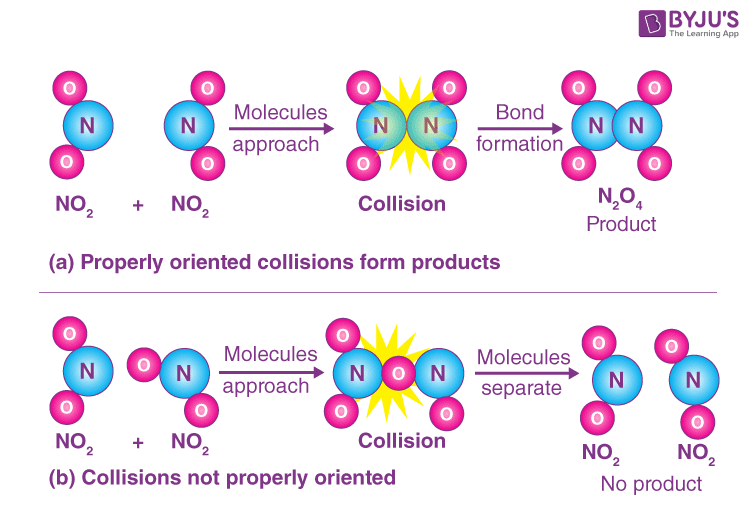Q2. What happens to the most probable kinetic energy and the energy of activation with increase in temperature?

The rise in temperature increases the average kinetic energy of the reacting species. The peak of the curve corresponds to the kinetic energy possessed by the maximum fraction of the molecule is known as the most probable kinetic energy. When the temperature is raised, the maximum of the curve moves towards higher energy. At that time, the fraction of the molecules with most probable kinetic energy decreases at higher temperatures.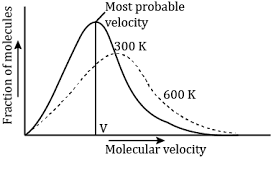Q3. Explain the difference between the instantaneous rate of a reaction and average rate of a reaction.

Average rate of a reaction is the change in molar concentration of the species taking part in a reaction divided by the time taken for the change. Mathematically,

rav = – Δ[R] /Δ[t] = Δ[P] / Δ[t]

Instantaneous rate of a reaction is the average rate at the smallest interval of time.

r(inst) = – d[R] /d[t] = d[P] / d[t]

Q4. With the help of an example explain what is meant by pseudo first order reaction

Consider the hydrolysis of ethyl acetate,The reaction can be represented as

CH3COOC2H5 + H2O → CH3COOH + C2H5OH

The rate equation can be given as,Rate = k [CH3COOC2H5] [H2O]

Here we see that the concentration of water is very high and thus does not change much during the course of the reaction. Thus the rate of the reaction can be said to be independent of the change in the concentration of H2O. Putting the term for change of concentration of water in the above reaction to being zero we can write the effective rate equation as,

Rate = k [CH3COOC2H5]

Here, the term takes into account the value of the constant concentration of water.

where K = K’ [H2O]

We see that the reaction behaves as a first-order reaction. Such reactions are termed as pseudo-first-order reactions.

Test your knowledge on Important Questions Class 12 Chemistry Chapter 4 Chemical Kinetics